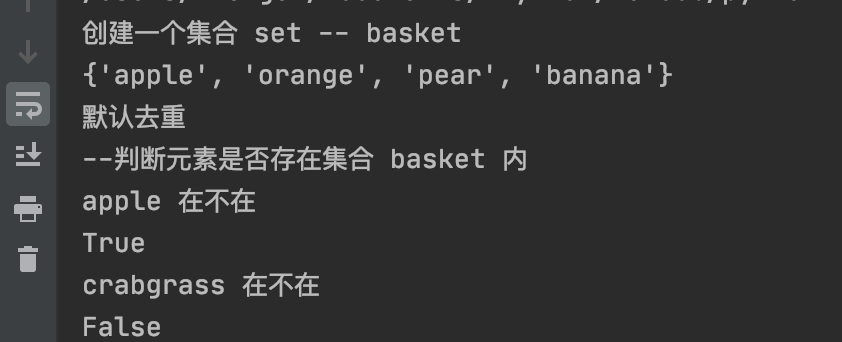1. ## Python基礎教程之Python 集合( Set)

2020-12-04 14:23發布

# Python  Set（集合）

集合（set）是由一個或數個形態各異的大小整體組成的，構成集合的事物或對象稱作元素或是成員。

基本功能是進行成員關系測試和刪除重復元素。

可以使用大括號 { } 或者 set() 函數創建集合，注意：創建一個空集合必須用 set() 而不是 { }，因為 { } 是用來創建一個空字典。

創建格式：

```parame = {value01,value02,...}
或者
set(value)```

## 創建實例

```dates = {'Number', 'String', 'List', 'Tuple', 'Set', 'List'}

print("輸出集合，重復的元素被自動去掉")
print(dates)```

與 java 類似，set 是無序且去重的數據結構

## 常用方法

包含關系

```if 'Set' in dates:
print("Set 在數據集合中")
else:
print("Set 不在數據集合中")```

集合運算

```# set可以進行集合運算
a = set('abc123')
b = set('123456')

print(a)
print(b)
print("a 和 b 的差集")
print(a - b)
print("a 和 b 的并集")
print(a | b)
print("a 和 b 的交集")
print(a & b)
print("a 和 b 中不同時存在的元素")
print(a ^ b)```

# Python3 集合

集合（set）是一個無序的不重復元素序列。

可以使用大括號 { } 或者 set() 函數創建集合，注意：創建一個空集合必須用 set() 而不是 { }，因為 { } 是用來創建一個空字典。

創建格式：

```parame = {value01,value02,...}
或者
set(value)```

實例(Python 3.0+)

上面已經提到過了，不在過多贅述

```print('創建一個集合 set -- basket')
basket = {'apple', 'orange', 'apple', 'pear', 'orange', 'banana'}
print('默認去重')
print('apple 在不在')
print('crabgrass 在不在')

結果：類似列表推導式，同樣集合支持集合推導式(Set comprehension):

```print('類似列表推導式，同樣集合支持集合推導式(Set comprehension):')
a = {x for x in 'abracadabra' if x not in 'abc'}
print(a)```

結果:

類似列表推導式，同樣集合支持集合推導式(Set comprehension):
{'r', 'd'}

## 集合的基本操作

### 1、添加元素

語法格式如下：

`s.add( x )`

將元素 x 添加到集合 s 中，如果元素已存在，則不進行任何操作。

實例(Python 3.0+)

```setTest = set(('a', 'b', 'c'))
print('原始集合：', setTest)
print('增加一個元素，baba', setTest)```

結果如下：還有一個方法，也可以添加元素，且參數可以是列表，元組，字典等，語法格式如下：

`s.update( x )`

x 可以有多個，用逗號分開。

```setTest.update({1, 2, 3})
print('繼續增加一個字典，123后：', setTest)
setTest.update([1, 2], [3, 4, 5])
print('繼續增加一個列表后：', setTest)```

這時候結果：### 2、移除元素

語法格式如下：

`s.remove( x )`

將元素 x 從集合 s 中移除，如果元素不存在，則會發生錯誤。

```setDel = {"Google", "Baidu", "SoSo"}
print("原始集合：", setDel)
setDel.remove('SoSo')
print('刪除 soso 后', setDel)
setDel.remove('ali')
print('不存在會發生錯誤')```

結果：

Traceback (most recent call last):
File "/setTest.py", line 58, in <module>
setDel.remove('ali')
KeyError: 'ali'

此外還有一個方法也是移除集合中的元素，且如果元素不存在，不會發生錯誤。格式如下所示：

`s.discard( x )`

實例(Python 3.0+)

```>>> thisset = set(("Google", "ali", "Taobao"))
>>> print(thisset)

我們也可以設置隨機刪除集合中的一個元素，語法格式如下：

`s.pop()`

栗子：

```print('隨機刪除一個元素：',setDel.pop())
print(setDel)```

結果：

隨機刪除一個元素： Baidu

多次執行測試結果都不一樣。

set 集合的 pop 方法會對集合進行無序的排列，然后將這個無序排列集合的左面第一個元素進行刪除。

### 3、計算集合元素個數

語法格式如下：

`len(s)`

計算集合 s 元素個數。

```>>> thisset = set(("Google", "123", "Taobao"))
>>> len(thisset)
3```

### 4、清空集合

語法格式如下：

`s.clear()`

清空集合 s。

```>>> thisset = set(("Google", "bba", "Taobao"))
>>> thisset.clear()
>>> print(thisset)
set()```

### 5、判斷元素是否在集合中存在

語法格式如下：

`x in s`

判斷元素 x 是否在集合 s 中，存在返回 True，不存在返回 False。

```>>> thisset = set(("Google", "11111", "Taobao"))
>>> "11111" in thisset
True